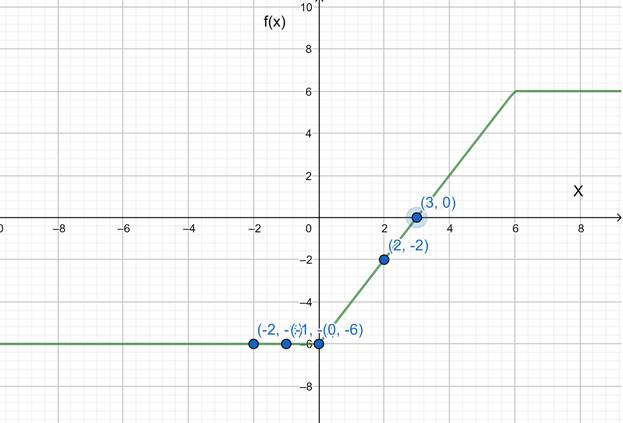# The graph of the function f ( x ) = | x | − | x − 6 | .### Precalculus: Mathematics for Calcu...

6th Edition
Stewart + 5 others
Publisher: Cengage Learning
ISBN: 9780840068071### Precalculus: Mathematics for Calcu...

6th Edition
Stewart + 5 others
Publisher: Cengage Learning
ISBN: 9780840068071

#### Solutions

Chapter 2.7, Problem 69E
To determine

## To sketch: The graph of the function f(x)=|x|−|x−6| .

Expert Solution

### Explanation of Solution

Given information: f(x)=|x||x6| .

Graph:

 x -2 -1 0 3 2 f(x) -6 -6 6 0 -2Interpretation:

The horizontal line test is a method that can be used to determine if a function is a one-to-one function. This means that, for every y-value in the function, there is only one unique x-value.

So, it is clear from the graph that the horizontal line cuts only once the graph.

Thus is a one-to-one graph.

### Have a homework question?

Subscribe to bartleby learn! Ask subject matter experts 30 homework questions each month. Plus, you’ll have access to millions of step-by-step textbook answers!